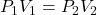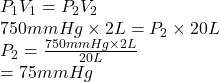2L of hydrogen has an initial pressure of 750 mmHg, what is the final pressure in mmHg if the volume increases to 20 L with a constant tempe

Question

2L of hydrogen has an initial pressure of 750 mmHg, what is the final pressure in mmHg if the volume increases to 20 L with a constant temperature of 37 degrees C?​

in progress 0
6 months 2021-08-16T04:53:50+00:00 1 Answers 0 views 0

1. Answer: The final pressure is 75 mm Hg.

Explanation:

According to Boyle’s law, at constant temperature the pressure of a gas in inversely proportional to volume.

Since, it is given that the temperature is constant. Hence, formula used is as follows.Substitute the values into above formula as follows.Thus, we can conclude that the final pressure is 75 mm Hg.